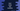# Dart program to swap two user given numbers## Dart program to swap two numbers:

This program will show how to swap two numbers in Dart. This is a beginner level program and with this program, you will learn how to take user inputs, how to store numbers in variables and how to swap two numbers using a third variable.

### Dart program:

In this program, we are swapping two int numbers by using a third variable. Below is the complete program.

``````import 'dart:io';

main(){
var firstNum, secondNum, temp;

stdout.write("Enter the first number : ");

stdout.write("Enter the second number : ");

temp = firstNum;
firstNum = secondNum;
secondNum = temp;

stdout.write("After swapping, first number : \$firstNum, second number : \$secondNum");
}``````

### Explanation:

In this program,

• firstNum, secondNum and temp are three variables to store the first number, second number and a temporary number.
• Using stdout.write, we are writing one message on the console asking the user to enter the first number.
• Using stdin.readLineSync(), we are reading the user input from the console and parsing that value using int.parse to integer. This integer value is stored in firstNum.
• Similarly, the second number is read and stored in secondNum.
• For swapping these numbers, we are using the temporary variable temp. It requires three steps to swap two numbers. First, assign the first number to the temporary variable. Second, assign the second number to the first number and finally assign the value in the temporary variable to the second number. It will swap the values store din the first and the second variable.
• The last line of the program prints the values of firstNum and secondNum.

### Output:

If you run this program, it will print the below output:

``````Enter the first number : 10
Enter the second number : 20

After swapping, first number : 20, second number : 10``````

As you can see, the numbers entered are swapped. You can try with different numbers to see different results.